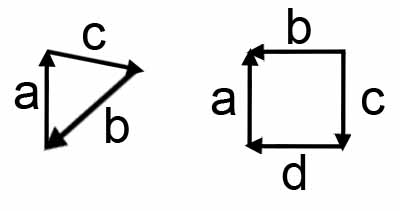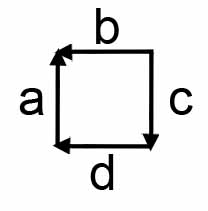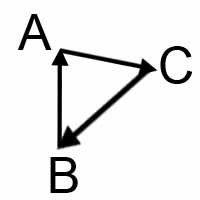# 4. Maths Vector Length

The first thing we will look at is how to work out the length of a vector.Take a look at the diagram and try to grasp that we can determine the length of every vector there.

————————Figure 1 – The triangle on the left———————-

First go to the iamge in the left. You will see a triangle with sides of length a, b and c.

The vector a is facing towards c, the vector b is facing towards a and the vector c is facing towards b.

So to work out the length of the vectors we start at the end of the vector WITHOUT the arrow and work our way around to the end with the arrow.

To work out the length of a, we would start at the end of a, so the start of b and work around to the end of a with the arrow. So our length would be:

a = -b + -c

Which comes down to:

a = -b-c

If we were to work out negative a, we would get:

-a = c + b

Because to work out the negative value, we start at the other end.

For our formulae for a to be:

a = -b-c

You have to take into account that moving in the same direction is positive and moving opposite to the arrow is negative.

So all our lengths for our triangle would be:

a = -b-c

b = -c-a

c = -a-b

————————Figure 2 – The square on the right———————-If you follow our rules from above, the lengths of our vectors come out to:

a = -d-c+b

b = c+d+a

c = b-a-d

d = -c+b-a

Keep in mind that no matter what shape you have, you ALWAYS work it out the same way. And thanks to vectors being what they are, this will always work.

# __LENGTH OF A VECTOR PART 2__

In this part, we are going to be looking at the length of a vector in almost the exact same way as before. Only this time, the characters, a,b and c are not lines, but points.To work out the length of these, you just have to work it out a little differently.

Take point A and point C. Now the length of that vector is AC (with a line above it)The arrow above it just represents a vector instead of a normal line.

So in this diagram we have lengths:

AC

CB

BA

And to work that out is exactly the same as before.

AC = |AC| or -|AB|-|BC|

Where the lines | | represent the length.

# __LENGTH OF A VECTOR PART 3__

Here we are going to be acctually calculating the length of the vector using numbers.A is going to be located at (1,1)

B is going to be located at (1,0)

C is going to be located at (2,0.5)

Now the line AC comes to:

AC = [cX – aX, cY – aY]

Therefore:

AC = [2 – 1, 0.5 – 1]

AC = [1, -0.5]

Now to work out the length/distance:

d = sqrt(x^2 + y^2)

And vectors dont acctually have a length measuring system like cm, m, km etc. They are just measured in units.

so AC = sqrt(1^2 + (-0.55)^2)

AC = sqrt(1 + 0.25)

AC = sqrt(1.25) units.

If you have any questions, please email me at swiftless@gmail.com

• March 25, 2010
• 1